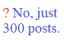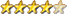I2C  SSD1306 0.9" LCD

Author Message
phil99GuruJoined: 11/02/2018
Location: Australia
Posts: 1483
 Posted: 12:10pm 26 Oct 2022

LOG() seems to work for me, ln e = 1.

> Print log(2.71828183)
1.000000001
>

Using
R1 = 10000 ' series resistor
beta = 4000  'thermistor coefficient
R0 = 10000   'thermistor reference resistance
T0 = 25   'thermistor reference temperature
Vs = 3.322   'Supply voltage
in this
Ra = R1 * Vin / (Vs - Vin )   ' Calculate resistance
Temp = 1/(1/(T0+273.15) + Log(Ra/R0)/beta)-273.15   ' Calculate temperature

I get
> R1 = 10000 : Vs = 3.322 : Vin = 1.661 : beta = 4000 : R0 = 10000 : T0 = 25
> Ra = R1 * Vin / (Vs - Vin )
> ? Ra
10000
> Temp = 1/(1/(T0+273.15) + Log(Ra/R0)/beta)-273.15
> ? Temp
25
>

Which is correct.